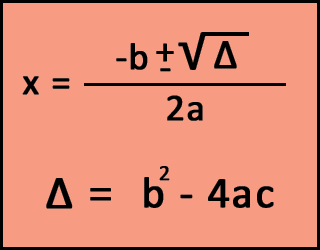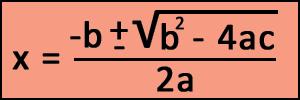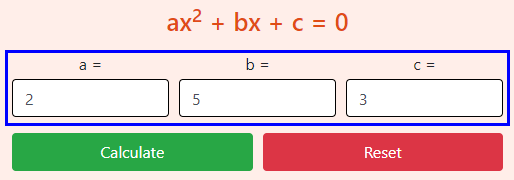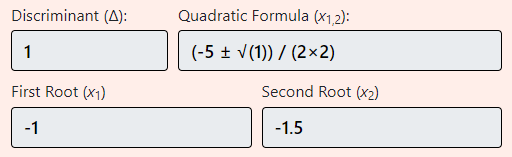# Quadratic Formula Calculator

Quadratic Formula Calculator. This free online tool helps you to solve your second-order polynomial quadratic equations.

### ax2 + bx + c = 0

##### More Useful Tools
Standard Deviation Calculator Fraction Calculator Z Score Calculator

## About Quadratic Formula Calculator• Do you ever wish for a Quadratic Formula Calculator that can make your complex calculations easier?
• Do you want to verify your results after solving a quadratic equation?

If so, then here it is the one-stop solution for solving discriminant, real and complex roots with formula. It's very easy to use and 100% free. Also, in this article, we will discuss the formula, symbols, and how we can determine the discriminant and roots.

### What is Quadratic Equation?

The Quadratic name comes from "Quad". Also, we can call it "Square" (Like x2). It is a solution of a second-order polynomial equation with three coefficients.

We can write the equation in standard form like this:

Ax2 + Bx + C = 0 ( Where A ≠ 0 )

Here, A, B, and C are the coefficients or we can call it "Known Numbers".
Whereas, the 'x' is the unknown variable that we need to find out using the Quadratic Formula.

The following are some examples for better understanding.

 3x2 + 4x + 1 = 0 Here a=3, b=4, and c=1 2x2 - 4x = 0 You can see there is just 2 coefficients values at left side. So, we can figure our the values like this:a = 2b = 4c = 0 (If there is no values then we can consider it 0) 4x + 2 = 0 It is not a quadratic equation. Because there is no x2 value. So, 'a' is counted as 0.

Sometimes the quadratic equations are not in standard form. So, we have to move or expand some coefficients to convert it into standard form.

Let's see some examples:

Disguise Form Rearrangements Standard Form Coefficients
2x2 = 5x - 4 Move right values to left side 2x2 - 5x + 4 = 0 a=2, b=-5, c=4
4(y2 + 3y) = 2 Expand left side and move 2 to left 4y2 + 12y - 2 = 0 a=4, b=12, c=-2

#### Quadratic Formula

The formula looks like below and we can use it to find the "x" value.Where,

• a, b, and c are the coefficients. We already have its values.
• Δ represents the Discriminant. It is used to check the nature of roots.
Δ = b2 - 4ac

Our quadratic equation solver determines the Discriminant values, whether it is greater than, less than, or equal to 0.

If b2 - 4ac is,

• Zero, then there is 1 real root.
• Positive, then there are 2 real root.
• Negative, then there are 2 complex roots.

### How to solve a Quadratic Equation?

There are various ways to calculate the discriminant and roots which are given below.

1. Factoring Quadratic
2. Completing the Square
3. Using Quadratic Formula

In this article, we will only discuss the third method. Which is using the quadratic formula. Also, it is the most straightforward way to find the roots. Even more, the quadratic equation calculator uses the same concept behind the hood.

Let's take some examples to understand the process step by step.

##### Example 1: Solve 2x2 + 5x = -3

First of all, we have to arrange the equation in standard form like this: ax2 + bx + c = 0.

Take -3 from right to left-hand side. As a result, we get:

2x2 + 5x + 3 = 0

Now look at the formula and figure out what things are known and unknown. Also, put them in their position.In the formula, you have to put a, b, and c in their respective places.

In our case, the values are:

• a = 2
• b = 5
• c = 3

After putting them in the formula, we get,

x = -5 ± √(52) - 4(2 x 3)
2 x 2

= -5 ± √25 - 24
4

= -5 ± 1
4

As you can see, the discriminant(b2 - 4ac) = 1 that is > 0. So, there are two real roots.

For the First Root:

x = -5 + 1
4

x = -1

For the Second Root:

x = -5 - 1
4

x = -1.5

Hence, the roots of 2x2 + 5x + 3 = 0 are -1 and -1.5.

##### Example 2: Solve 4x2 + 15x + 20 = 0

Here Coefficients are: a = 4, b = 15, c = 20.

So, first of all, let's find the discriminant:

Δ = b2 − 4ac

= 152 - 4(4 x 20)

= 152 - 4(80)

= 225 - 320

= -95

Note that, the discriminant is -95. That is negative. So, we will get 2 complex roots.

Now let's find the roots.

x = -15 ± √(-95)
2 x 4

x = -15 ± √(-95)
8

√(-95) = 1.21835i
(i = √−1, Imaginary number)

So, x = -15 ± 1.21835i
8

Final Answer: -1.875 ± 1.21835i

#### Linear Equation

A linear equation has only one solution or root. In the standard form of quadratic equation, if 'a' becomes 0, then the equation evaluates to bx + c = 0. Hence, it becomes a linear equation. The solution of a linear equation is given by:

• bx + c = 0
• bx = -c
• x = -c/b

### Graphical Representation

The graph of a quadratic equation is basically a U-shaped curve called Parabola. The shape of the curve depends upon the sign of 'A' (the coefficient with x2).

• If a > 0 then the parabola goes up (like a smiley face).
• If a < 0 then the parabola goes down (like a sad face).

#### Features of Quadratic Equation Solver

• We have designed a very simple user interface of the Quadratic Equation Calculator. So, anyone can easily go through the calculator and find roots easily.
• The calculator also accepts decimal and integer(positive and negative) inputs.
• Along with the roots, you can also know the value of Discriminant. Hence, it also works as a Discriminant Calculator.
• Even more, it gives you a full formula as an output with entered values.

### Advantages of Quadratic Formula Calculator

• Determining roots using the formula looks simple for easy equations. Whereas, for complex values, it will take a lot of time as well as effort. Therefore, the Quadratic Formula Calculator is the best option. Just fill the coefficients and sit back, relax, and get the roots.
• Manually finding the roots is not 100% accurate way. You may sometimes get the wrong results. However, the calculator never returns an incorrect result. So, use the Quadratic Equation Solver to get 100% accurate results.
• Another big advantage is that you can use it anytime anywhere. Just you need the Internet connection and a smart device to access the calculator.
• You can use this as a way to verify your roots. It can help in your mathematics studies. Also, you can use it for self-assessment.

#### How to use Quadratic Formula Calculator?

The process is very simple. But you need a smart device like a computer, smartphone, or iPad with a browser installed. Also, your device must be connected with the Internet. Because this is an online calculator. So, the internet is compulsory.

After that, open the Quadratic Formula Calculator on your device browser and follow the instructions below.

##### 1. Arrange Equation in Standard Form

First of all, arrange your equation in the standard form.

For example, if your equation is:
6 + 3x2 + 2x = -3.

Then it should be rearranged to:
3x2 + 2x + 9 = 0

##### 2. Enter Coefficients

When you open the calculator, you can see there are three input boxes. These fields are for entering the values of the coefficients. The first one is for A value, second is for B value, and the third is for C value. So, enter your values as per your requirements.##### 3. Calculate or Reset

After filling coefficients, just press the Calculate button to start the calculation. For new calculations, you can use "Reset" button.##### 4. Get Output

Finally, you will get Discriminant, First root, and Second Root as an output. Also, our calculator gives you the Quadratic Formula with entered values.### Conclusion

Using our online Quadratic Formula Calculator, you can easily determine the quadratic equations and roots. Also, you can learn so many things from this article with examples. Most importantly, it will give you the final results in just a few keystrokes.